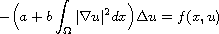Electron. J. Diff. Equ., Vol. 2013 (2013), No. 257, pp. 1-8.

### Ground state solution of a nonlocal boundary-value problem Cyril Joel Batkam

Abstract:
In this article, we apply the Nehari manifold method to study the Kirchhoff type equationsubject to Dirichlet boundary conditions. Under a general 4-superlinear condition on the nonlinearity f, we prove the existence of a ground state solution, that is a nontrivial solution which has least energy among the set of nontrivial solutions. If f is odd with respect to the second variable, we also obtain the existence of infinitely many solutions. Under our assumptions the Nehari manifold does not need to be of class C^1.

Submitted June 24, 2013. Published November 22, 2013.
Math Subject Classifications: 35J60, 35J25.
Key Words: Nonlocal problem; Kirchhoff's equation; ground state solution; Nehari manifold.

Show me the PDF file (214 KB), TEX file, and other files for this article.Cyril Joel Batkam Département de mathématiques Université de Sherbrooke Sherbrooke, Québec, J1K 2R1, Canada email: cyril.joel.batkam@usherbrooke.ca